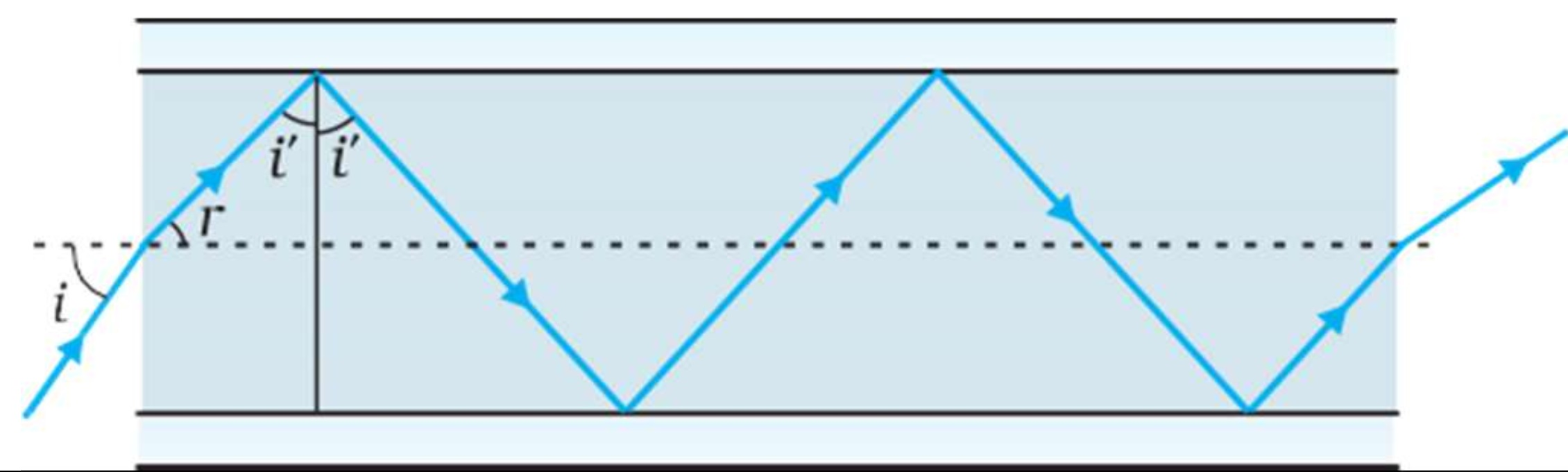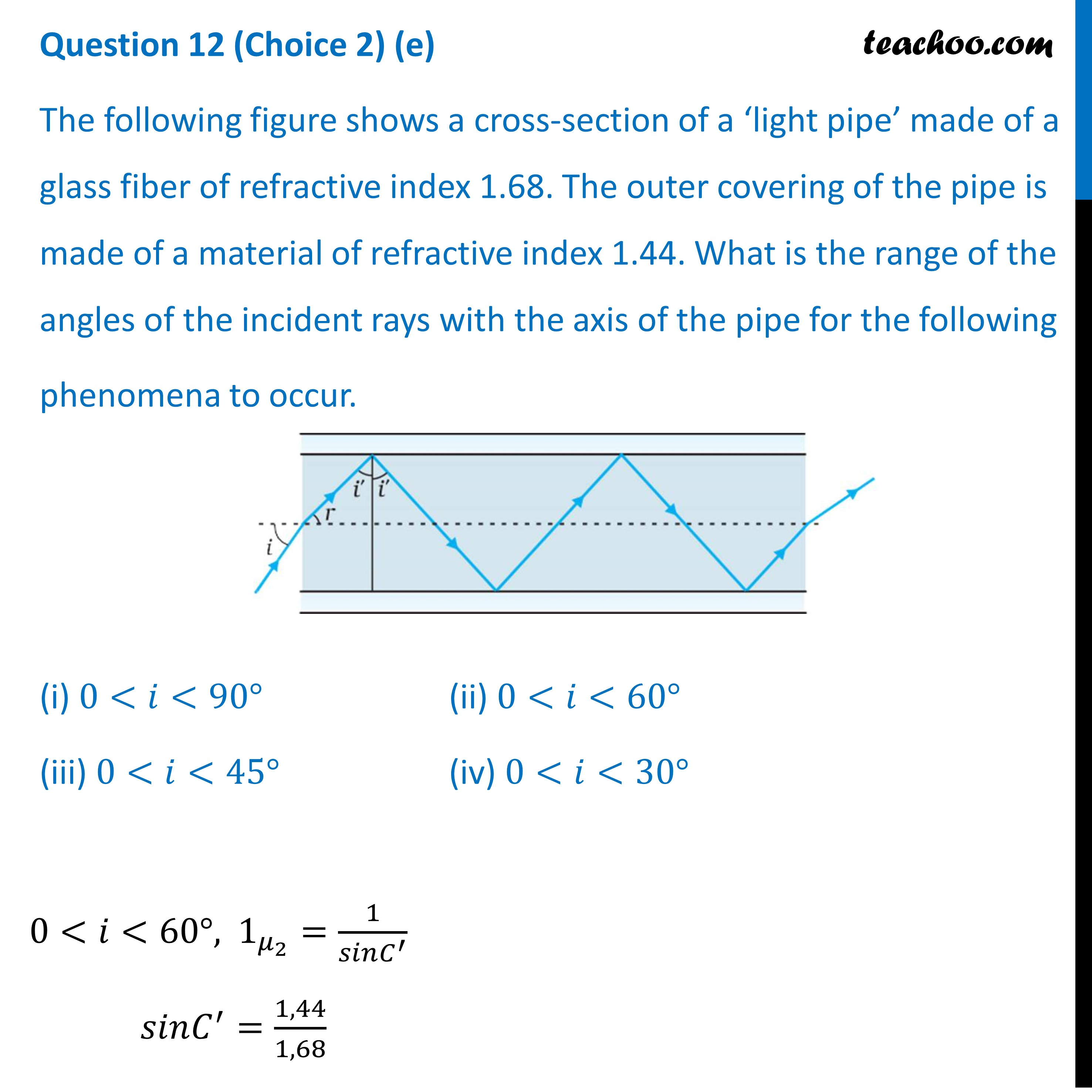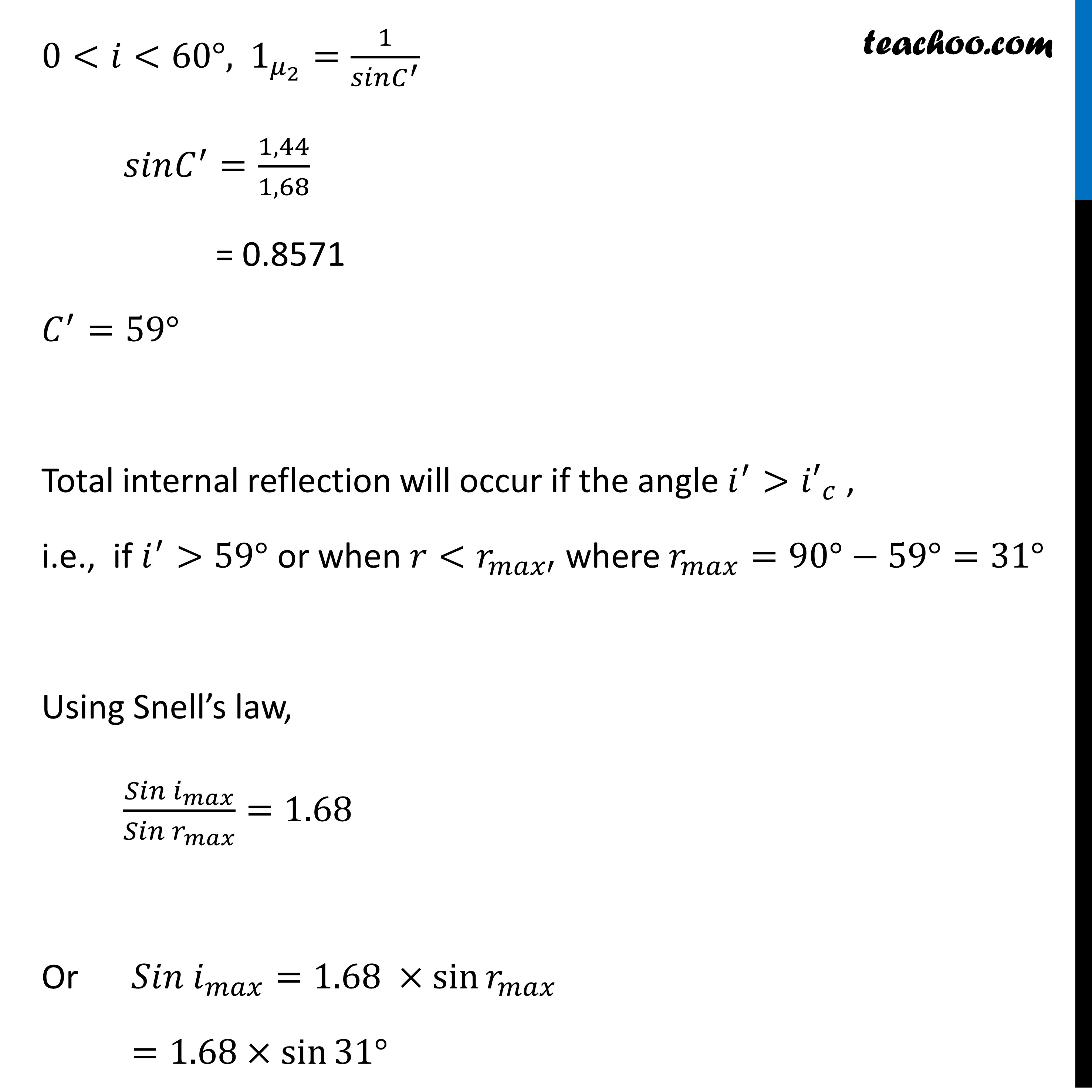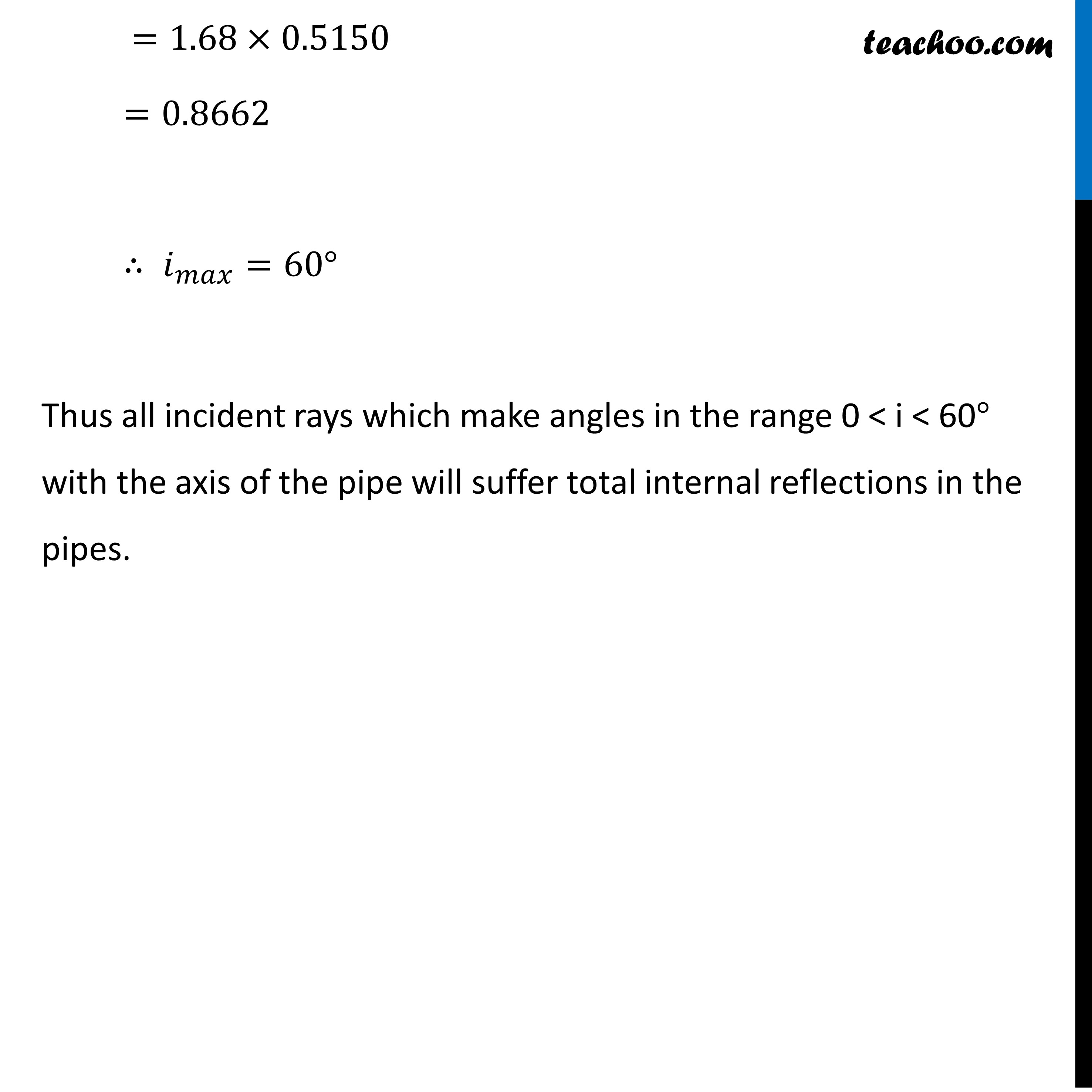CBSE Class 12 Sample Paper for 2022 Boards [Term 2] - Physics

Physics Class 12
Solutions to CBSE Sample Paper - Physics Class 12

## The following figure shows a cross-section of a ‘light pipe’ made of a glass fiber of refractive index 1.68. The outer covering of the pipe is made of a material of refractive index 1.44. What is the range of the angles of the incident rays with the axis of the pipe for the following phenomena to occur.## (iv) 0 < 𝑖 < 30°Learn in your speed, with individual attention - Teachoo Maths 1-on-1 Class

### Transcript

Question 12 (Choice 2) (e) The following figure shows a cross-section of a ‘light pipe’ made of a glass fiber of refractive index 1.68. The outer covering of the pipe is made of a material of refractive index 1.44. What is the range of the angles of the incident rays with the axis of the pipe for the following phenomena to occur. (i) 0<𝑖<90° (ii) 0<𝑖<60° (iii) 0<𝑖<45° (iv) 0<𝑖<30° 0<𝑖<60°, 1_(𝜇_2 )=1/(𝑠𝑖𝑛𝐶^′ ) 𝑠𝑖𝑛𝐶^′=1,44/1,68 0<𝑖<60°, 1_(𝜇_2 )=1/(𝑠𝑖𝑛𝐶^′ ) 𝑠𝑖𝑛𝐶^′=1,44/1,68 = 0.8571 𝐶^′=59° Total internal reflection will occur if the angle 𝑖^′>〖𝑖′〗_𝑐 , i.e., if 𝑖^′>59° or when 𝑟<𝑟_𝑚𝑎𝑥′ where 𝑟_𝑚𝑎𝑥=90°−59°=31° Using Snell’s law, (𝑆𝑖𝑛 𝑖_𝑚𝑎𝑥)/(𝑆𝑖𝑛 𝑟_𝑚𝑎𝑥 )=1.68 Or 𝑆𝑖𝑛 𝑖_𝑚𝑎𝑥=1.68 ×sin⁡〖𝑟_𝑚𝑎𝑥 〗 =1.68×sin⁡〖31°〗 =1.68×0.5150 =0.8662 ∴ 𝑖_𝑚𝑎𝑥=60° Thus all incident rays which make angles in the range 0 < i < 60° with the axis of the pipe will suffer total internal reflections in the pipes.